## General Question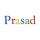# How far is the horizon?

Asked by prasad (3859) July 20th, 2012

Assuming everything is flat (or more precisely visible) around, how far is the horizon from where I stand? Approximately?

I know, it can be mathematically calculated, but I’m just lazy now.

And, how much area would that be that I can see all around me 360 degrees? What is the shape of the area called? I imagine the shape like cornea of the human eye or a contact lens.

Observing members: 0Composing members: 0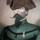I think I heard once that it’s about 20 miles, if you’re in Kansas or the desert-y part of Australia.
Horizon calculator here.

Nullo (21978)“Great Answer” (1) Flag as…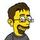Variable. I am six feet tall, so for me, the horizon is a little further away than it is for my wife who is 5’5”.

But there are calculators to figure it out for you.

That figures out to about 3 miles for an average person standing on the ground, or about 12 miles for someone standing ~100 feet off the ground.

jerv (31061)“Great Answer” (2) Flag as…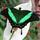I’m about 5’10”, so I’m 2.7 miles from where I see the horizon.
Now it says that I’m 3 miles from there, so my horizontal vision has gained a few yards.

Brian1946 (29161)“Great Answer” (1) Flag as…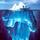What the calculators don’t show is a simple approximation formula, given by Wikipedia here, where distance to horizon is proportional to square root of height. Excerpt:
———————————-
Distance to the horizon
Ignoring the effect of atmospheric refraction, distance to the horizon from an observer close to the Earth’s surface is about
d ≈ 3.57 √h
where d is in kilometres and h is height above ground level in metres.

Examples, assuming no refraction:

For an observer standing on the ground with h = 1.70 metres (5 ft 7 in) (average eye-level height), the horizon is at a distance of 4.7 kilometres (2.9 mi).
For an observer standing on the ground with h = 2 metres (6 ft 7 in), the horizon is at a distance of 5 kilometres (3.1 mi).
For an observer standing on a hill or tower of 100 metres (330 ft) in height, the horizon is at a distance of 39 kilometres (24 mi).
For an observer standing at the top of the Burj Khalifa (828 metres (2,717 ft) in height), the horizon is at a distance of 111 kilometres (69 mi).

With d in miles and h in feet,
d ≈ 1.22 √h

Examples:

For an observer standing on a hill or tower 100 feet (30 m) in height, the horizon is at a distance of 12.2 miles (19.6 km).
For an observer on the summit of Aconcagua (22,841 feet (6,962 m) in height), the sea-level horizon to the west is at a distance of 184 miles (296 km).

gasman (11315)“Great Answer” (8) Flag as…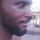As far as I know the horizon is that indefinite observable distance.. so give it a measurement it would mean it is no longer indefinite, thus ceasing to be the horizon but a definite point out in the distance.

kess (3917)“Great Answer” (6) Flag as…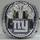I saw a man pursuing the horizon;

Round and round they sped.

I was disturbed at this;

I accosted the man.

“It is futile,” I said,

“You can never —”

“You lie,” he cried,

And ran on.

—-Stephen Crane

bkcunningham (18491)“Great Answer” (1) Flag as…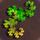On a perfectly flat plane, the horizon appears to be at exactly eye level and is an infinite distance away.

On a perfect sphere, the horizon is a finite distance away and depends on the diameter of the sphere and the height of the observer on the sphere. In order to find the distance to the horizon, you can use trigonometry and other geometry.

Imagine a triangle OCH, for observer-center-horizon. C is located at the center of the sphere, and H is the point on the sphere that appears as the horizon.

It should be easy to see that the visual line of sight (line OH) will be tangent to the sphere, since it can’t intersect the sphere and can’t be looking away from the sphere. Line CH is a radius of the sphere. These two facts combined mean that angle H is a right angle, so triangle OCH is a right triangle.

If the radius of the sphere (CH) is r, and the height of the observer is s, then the length of OC is equal to r + s.

By the Pythagorean theorem, (OH) = sqrt( (CH)^2 + (OC)^2 )
OH = sqrt(r^2 + (r+s)^2) = sqrt( r^2 + r^2 + 2rs + s^2)
This gives the length of the line of sight to the horizon

To find the distance to the horizon following the curve of the sphere, you need trigonometry.
This law of sines states that
OH/sin( C ) = CH/sin(O) = OC/sin(H)

We want to find angle C.

OC = r+s
H = 90
sin(90) = 1 {this makes the problem a lot easier}

OC/sin(90) = r+s = OH/sin( C )
OH = r
r+s = r / sin( C )
sin( C ) = r / (r+s)
C = sin^-1(r / (r+s)) #inverse sine

Now that we know angle C and that the circumference of the sphere is 2*r*pi, we can find the portion of the circumference that is the distance from the observer to the horizon.

Distance = 2*r*pi / 360 * sin^-1(r / (r+s))
where r = radius of Earth (or any sphere) and s = height of observer off the ground

PhiNotPi (12677)“Great Answer” (2) Flag as…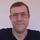Don’t forget that the Earth is a potato, not a perfect sphere. So even on the ocean the calculator won’t work perfectly. Gravity is not homogenous and does vary.

mattbrowne (31714)“Great Answer” (2) Flag as…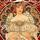@PhiNotPi I wonder if Chris Columbus knew all that math before he went road trippin’!

Dutchess_III (45313)“Great Answer” (0) Flag as…or